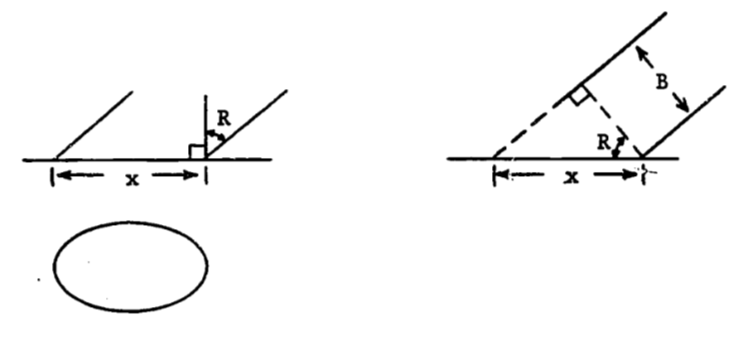## 1.12 Solution to the Additional Problems

1. First partial derivatives: \begin{align*} \frac{\partial E^2}{\partial A} &= \int^{30}_3\frac{\partial}{\partial A}(6.1(x+5.0)e^{-0.045x}-Ax-B)^2dx \\ &= 2 \int^{30}_3(6.1(x+5.0)e^{-0.045x}-Ax-B)(-x)dx \end{align*} Equating to zero and dividing by 2 gives $0 = -\int^{30}_36.1(x-2+5x)e^{-0.045x}dx +\int^{30}_3Ax^2+Bxdx$ Successive integration by parts (for the first integral) and direct integration (for the second integral) yield the first equation: $0 = 8991.0A + 445.5B - 25984.9$ \begin{align*} \frac{\partial E^2}{\partial B} &= \int^{30}_3\frac{\partial}{\partial B}(6.1(x+5.0)e^{-0.045x}-Ax-B)^2dx \\ &= -2 \int^{30}_3(6.1(x+5.0)e^{-0.045x}-Ax-B)dx \end{align*}

Equating to zero, dividing by 2 and solving yields the second equation: $0 = 445.5A + 27.0B - 1568.8$

The solutions are $$A= 0.061$$, $$B = 57.1$$. The least squares integral using the approximation $$Y = 0.061x + 57.1$$ is 546.452.

1. Let $$x=a\sin\theta$$. The limits of integration are then changed as follows $-a \le x \le a$ $-a \le a\sin\theta \le a$ $-1 \le \sin\theta \le 1$

There are many options for the range of $$\theta$$. We first change the integrand to see which range is suitable. We know the integrand is non-negative since $$x^2 \le a^2$$. Substitution gives $x = a\sin\theta$ $dx = a\cos\theta\:d\theta$ $\sqrt{a^2-x^2}dx = \sqrt{a^2 - a^2\sin^2\theta}\cdot a\cos\theta \:d\theta$ The integrand is then non-negative when the positive square root is used and when $$\cos \theta$$ is non-negative. Thus, we choose the limits of integration to be $$-\frac{\pi}{2}$$, $$\frac{\pi}{2}$$ since $$\sin(-\frac{\pi}{2}) = 1, \sin(\frac{\pi}{2}=1)$$ and $$\cos\theta \le0$$ if $$-\frac{\pi}{2} \le \theta \le \frac{\pi}{2}$$.
The integral is then $\int^{\pi/2}_{-\pi/2}\sqrt{a^2-a^2\sin^2\theta}a\cos\theta \:d\theta = a^2\int^{\pi/2}_{-\pi/2}\sqrt{1-\sin^2\theta}\cos\theta\:d\theta$ \begin{align*} &= a^2\int^{\pi/2}_{-\pi/2}\cos^2\theta\:d\theta=a^2[\frac{\theta}{2}+\frac{\sin2\theta}{4}]^{\pi/2}_{-\pi/2} \\ &= a^2[\pi/4+(1/4)(0)-(-\pi/4)-(1/4)(0)] =\pi a^2/2 \end{align*} Thus $A=\frac{2b}{a}\int^a_{-a}\sqrt{a^2-x^2}dx = \pi ab$ When $$a = b = 5.57$$, $$A = 97.47$$.
When $$a = 5$$, $$b = 6.09$$, $$A = 95.66$$.From the right-hand diagram, we have $\cos R = B/x$ and thus $x=B/\cos R$ The minor axis is then the circle’s diameter, $$B$$. In the answer to part “a”, we replace $$2a$$ by $$B/\cos R$$ and $$2b$$ by $$B$$.

\begin{align*} A &= \pi ab = \pi(B/2\cos R)(B/2) \\ &= \pi B^2 / (4 \cos R) \end{align*}

Since the area of the circle is $$\pi B^2/4$$,the dilution coefficient is $D = \frac{\pi B^2/4}{\pi B^2/4\cos R} = \cos R$ Thus $$D=0.6$$ means less dilution than $$D=0.3$$, and $$D=1.0$$ means no dilution at all. For Minnesota, the coefficients are
$$D=0.94$$ for $$R=20°$$
$$D=0.42$$ for $$R=65°$$